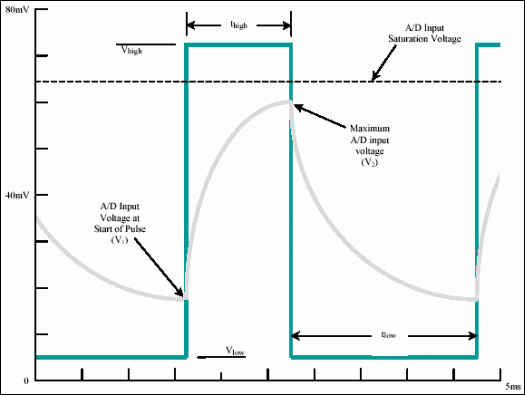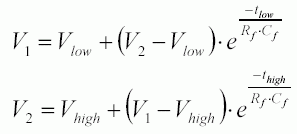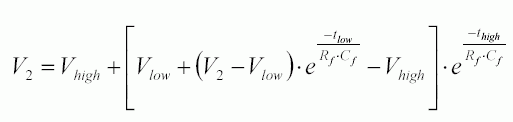# Increasing the Fuel Gauge Dynamic Range

### Abstract

The DS27xx series of fuel gauge devices from Maxim all specify a dynamic range over which a constant current can be measured. However, peak currents higher than this range can be measured by implementing a RC filter that can smooth the waveform into the measurable range of the device. This application note discusses how to implement this filter using a single external capacitor and then proceeds to instruct the reader on how to choose the proper capacitor value for the application.

### IntroductionFigure 1. DS27XX A/D Filter Circuit.

The data sheet specified maximum allowable input range for the current sensing A/D on DS27XX fuel gauge devices is displayed as a constant current value. This is not the true peak current measurable however. The A/D input of every Maxim fuel gauge contains an RC filter that will smooth the waveform and allow peaks greater than the specified maximum to be properly integrated. The filter capacitance is selectable to produce a waveform that uses the full range of the A/D but does not saturate its input.

### OperationFigure 2. Filtered and unfiltered waveforms.

The filter resistance is internal to the device and cannot be altered; the filter capacitor is connected between the IS1 and IS2 inputs. A value for the filter capacitor should be chosen such that the full range of the A/D is utilized without exceeding the input limit. The example in Figure 2 shows an original waveform greater than the A/D input range and the filtered waveform with a maximum peak (V2) less than the A/D input range.

The ideal value for the filter capacitor can either be determined experimentally or mathematically. The maximum filtered voltage can be represented in terms of the filter components and input waveform parameters. Equation 1 shows how the maximum filtered voltage is derived assuming the pattern is repetitive and the filtered waveform has reached a steady state. From this equation a capacitor value can be selected that meets the requirements of the application. The derivation of Equation 1 is shown in the appendix.Equation 1. Calculation of the maximum filtered voltage

Where:

 V1 = Minimum voltage of the filtered waveform V2 = Maximum voltage of the filtered waveform Vlow = Minimum voltage of the input waveform Vhigh = Maximum voltage of the input waveform tlow = The low period of the input waveform thigh = The high period of the input waveform Rf = The filter resistance inside the DS27XX device Cf = The selected external filter capacitance

For example, if a DS2762 with a filter resistance (Rf) of 9.4kΩ (two 4.7kΩ resistors in series) and input voltage limit of 64mV is used to measure the waveform in Figure 1 (Vlow = 6mV, Vhigh = 74mV, tlow = 2.0ms, thigh = 1.125ms), a filter capacitance (Cf) of at least .068µF is needed to prevent A/D input saturation. This calculation does not include device or waveform tolerances. Assume a filter resistance tolerance of ±15% of the listed datasheet value.

### Summary

DS27XX-based fuel gauges can measure current pulses much greater than their specified input range by filtering the waveform to be measured. The shape of the waveform is altered, changing individual current measurements, but the waveform energy is maintained keeping the accumulated current measurement accurate.

### Appendix - Cf Calculation Equations

Given the input waveform in Figure 1, the maximum voltage seen by the Current A/D can be calculated mathematically. For the filtered waveform to be at a steady state, the voltage increase during the high period of the pulse must equal the voltage decrease during the low period of the pulse. The filtered peak voltage can therefore be related to the filtered minimum voltage by the following equations:Where:

 V1 = Minimum voltage of the filtered waveform V2 = Maximum voltage of the filtered waveform Vlow = Minimum voltage of the input waveform Vhigh = Maximum voltage of the input waveform tlow = The low period of the input waveform thigh = The high period of the input waveform Rf = The filter resistance inside the DS27XX device Cf = The selected external filter capacitance

Substituting for V1 by combining the two equations produces:Which can then be reduced to:Solving for Cf beyond this point becomes mathematically complex. An iterative process can be used to determine a value for Cf that limits the maximum voltage V2 to below the saturation point of the A/D. Remember to include device tolerances when calculating a value for Cf.